Our World Statistics Day conversations have been a great reminder of how much statistics can inform our lives. Do you have an example of how statistics has made a difference in your life? Share your story with the Community!
Choose Language Hide Translation Bargail_massariCommunity Manager
More choices for modeling nonlinear responses

Mark Bailey (@markbailey) Principal Analytical Training Consultant, Education and Training, SAS

Clay Barker (@clay_barker) JMP Senior Research Statistician Developer, SAS

NOTE: This article originally appeared in JMPer Cable, Issue 28, Summer 2013. It has been updated to reflect changes made to JMP since 2013.

There are many instances where the response to a change in a variable is linear. In other cases, the response is not linear. A nonlinear response can sometimes be approximated by a model that is linear in the parameters (linear models). These models are a linear combination of terms in which the parameters appear singly in each term and only as a coefficient. A common linear model for a nonlinear response is a polynomial function, such as:

y = β0 + β1x1 + β2 x2 + β12 x1x2 + β11x12 + β22 x22.

Finally, other cases of nonlinear responses require a model that is nonlinear in the parameters (nonlinear models). There are myriad such models, for example  y = α + β e−γ x , that are used across a broad range of disciplines.

Let’s watch as the Fit Curve platform handles two common situations:

• Determining expiry (shelf life) with an exponential decay
• Comparing a sample to a standard with a logistic calibration curves as used in bioassays or immunoassays.

The Chlorine degradation data table (shown here) contains measurements of the chlorine level of 42 batches of a disinfectant at various ages (days since preparation). You need to determine when the level falls below the lower specification level of 0.4 (LSL).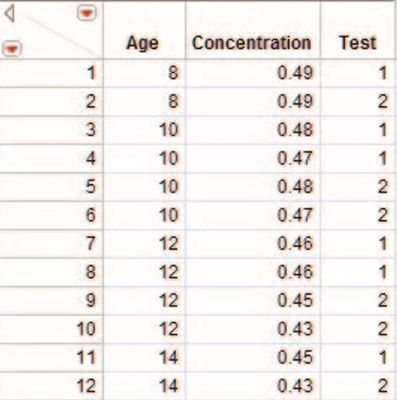1. Select Analyze > Specialized Modeling > Fit Curve.
2. Select Concentration and click Y, Response.
3. Select Age, click X, Regressor, then click OK.

The decrease in the chlorine level over time is apparent from the scatterplot (Figure 1).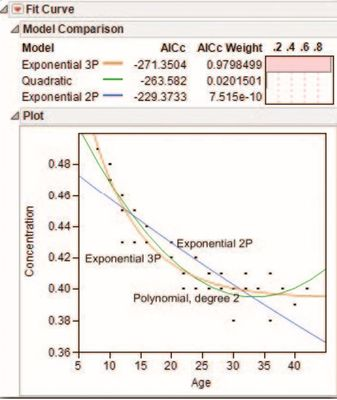Figure 1 Scatterplot with exploratory fits

You can explore the shape of the data using commands in the red triangle menu on the Fit Curve title bar. The change is not linear, so start with a quadratic polynomial.

1. Select Polynomials > Fit Quadratic.

This model captures the curvature, but the parabolic shape must turn up as Age increases past the minimum point, but the chlorine level does not rise in the future. Next try a simple growth (decay) model.

1. Select Exponential Growth and Decay > Fit Exponential 2P.

This curve is forced to zero as Age goes to infinity – it performs worse than the polynomial. Now use a growth curve with a non-zero asymptote.

1. Select Exponential Growth and Decay > Fit Exponential 3P.

This choice provides a reasonable model.

### Compare models

The Model Comparison report (Figure 2) provides essential performance indicators. The model list is sorted by AICc. The best model has the smallest AICc. Note that the second-best model has an AICc that is 7.8 higher, which indicates considerably less support from the data.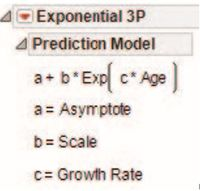Figure 2 Model Comparison reports

The Prediction Model reports beneath the scatterplot (shown here) provide the form of the model and the interpretation of the parameters for each model fitted to the data.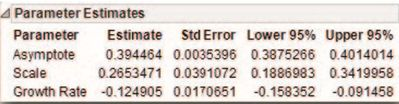The asymptote indicates that the degradation stops near the given LSL (lower specification level) of 0.40. The negative growth rate indicates that the decay is 0.125 units per day. Note: The sum of the scale and the asymptote indicates the starting level, 0.394464+0.2653471=0.6598111.

### Inverse prediction

You can perform an inverse prediction to determine expiry. Select Custom Inverse Prediction from the red triangle on the Exponential 3P title bar. Use 0.95 for the confidence level. Select Lower One Sided from the drop-down menu, enter 0.40 for Concentration and click OK. Figure 3 shows the fitted decay curve and the point estimate. The lower 95% confidence bound suggests that the disinfectant must be discarded and remade after 26 days.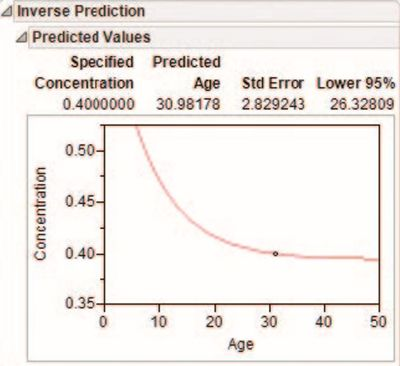Figure 3 Inversion Prediction Plot to estimate expiry

### Multiple samples

Now watch the Fit Curve platform in JMP use special features in a dose-response curve analysis. The Standard & Sample Dose-Response Analysis data table. Figure 4 includes an indicator (Sample) for the type of sample (“Standard” or Sample”), the concentration (Conc (ug/mL)) and log concentration (Log Conc), three standardized replicate assays, and the mean assay (Mean Assay).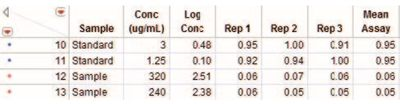Figure 4 Partial listing of the Dose-Response Analysis data table

1. Select Analyze > Specialized Modeling > Fit Curve.
2. Select Mean Assay and click Y, Response.
3. Select Log Conc and click X, Regressor.
4. Select Sample, click Group, then click OK.

You see in Figure 5 that data for both samples are plotted together and individually (blue is “Standard,” red is “Sample”).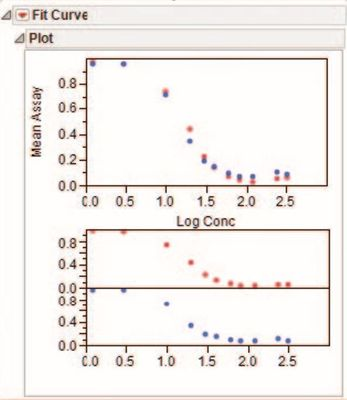Figure 5 Total and group plots for Mean Assay by Log Conc

Now Fit the data using a logistic model:

1. Select Sigmoid Curves > Logistic Curves > Fit Logistic 3P from the red triangle on the Fit Curve title bar.

This model accounts for both asymptotes, which is not possible with a polynomial model. Consider a more complex model:

1. Select Sigmoid Curves > Logistic Curves > Fit Logistic 4P from the red triangle on the Fit Curve title bar.

The additional flexibility afforded by this model fits the data better.

The addition of yet another parameter allows for asymmetry in the asymptotes:

1. Select Sigmoid Curves > Logistic Curves > Fit Logistic 5P from the red triangle on the Fit Curve title bar.

Look at the Model Comparison table in Figure 6 to see that the best model is Logistic 4P. The Logistic 3P model has essentially no support from the data (delta AICc is 92.80 – 60.38 = 32.42). The more flexible Logistic 5P model does not offer any real improvement. The AICc Weight helps with model selection (Figure 6). You may interpret this case to say that, given one of the three fitted models is true, then there is a 83% chance that the Logistic 4P is the true model (AICcWeight = .8259).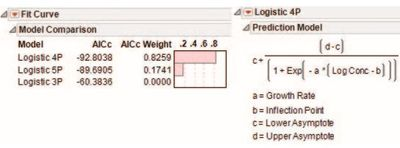Figure 6 AICc results for Logistic 4P model

Since the Sample variable is a Group variable in the analysis, there are separate sets of parameter estimates for each group.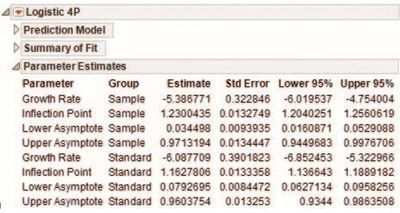Figure 7 Parameter estimates for each group

Figure 8 shows the curves based on the fitted model plotted together and separately for each group. It appears that the lower asymptote is different for the sample than it is for the standard.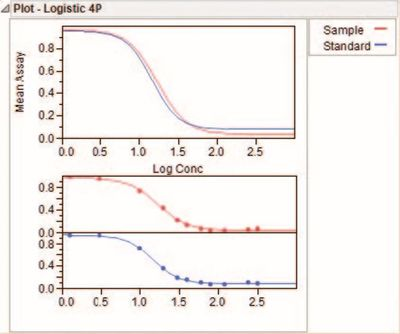Figure 8 Fitted curves for total Mean Assay and Sample Groups

The sample and standard curve appear to have a similar shape. If so, the relative potency can be estimated. To do this, select Test Parallelism from the red triangle menu on the Logistic 4P title bar.

Two hypothesis tests are provided. Both tests compare the full model to the reduced model. The full model estimates each parameter separately for the sample and the standard.

The reduced model estimates each parameter for the combined data, except the inflection point. In this example, the F-test is significant (0.0219) but the χ2-test is not at α=0.05. The F-test result might be a type I error, or it might indicate that the lower asymptote is not the same for the sample and the standard.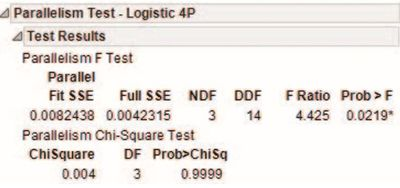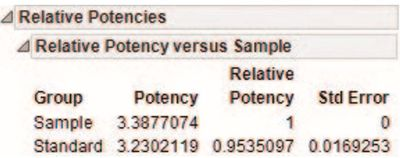The relative potency may be used when the curves are judged to be parallel. In this case, the potency of the sample is similar to that of the standard. The potency is the log concentration corresponding to the EC50 response.

JMP offers a newer approach to deciding about the relative shape in general, and parallelism in particular, that is based on an equivalence test for each parameter.

The newer approach is based on the two one-sided t=tests (TOST) approach, but it is presented graphically with confidence intervals for easier interpretation. The confidence interval for the ratio of the shape parameter estimates for the sample and the standard should be within a range deemed to be equivalent. The inflection point parameter may exceed this range, indicating a change in the relative potency.

To see this approach:

1. Select Equivalence Test from the red triangle menu on the Logistic 4P title bar.
2. Select “Standard” as the reference group and then click OK.

The two one-sided t=tests determine if the parameter estimates exceed a default 25 percent difference by ratio.  It is apparent that the lower asymptote is not equivalent with the standard, so these curves are not parallel (Figure 9). This is confirmed by the Equivalence Summary Table.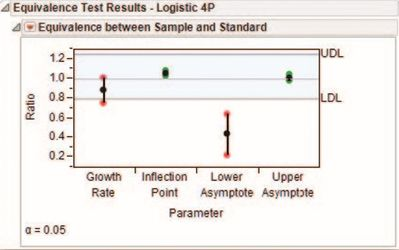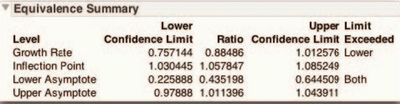Figure 9 Graphical Results of TOST Equivalence Test

### References

Burnham, Kenneth P., and Anderson, David R. (2002) Model Selection and Multimodel Inference: A Practical Information-Theoretic Approach (Second Edition), Springer, New York, NY, page 70.

SAS Institute Inc. (2012), Modeling and Multivariate Methods, Cary, NC: SAS Institute Inc.

Article Labels
Article Tags